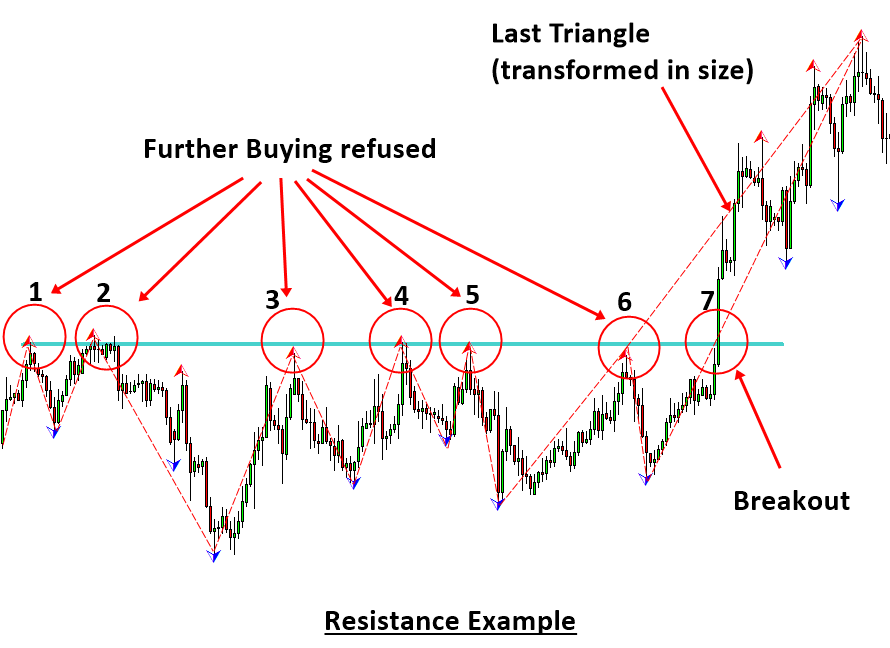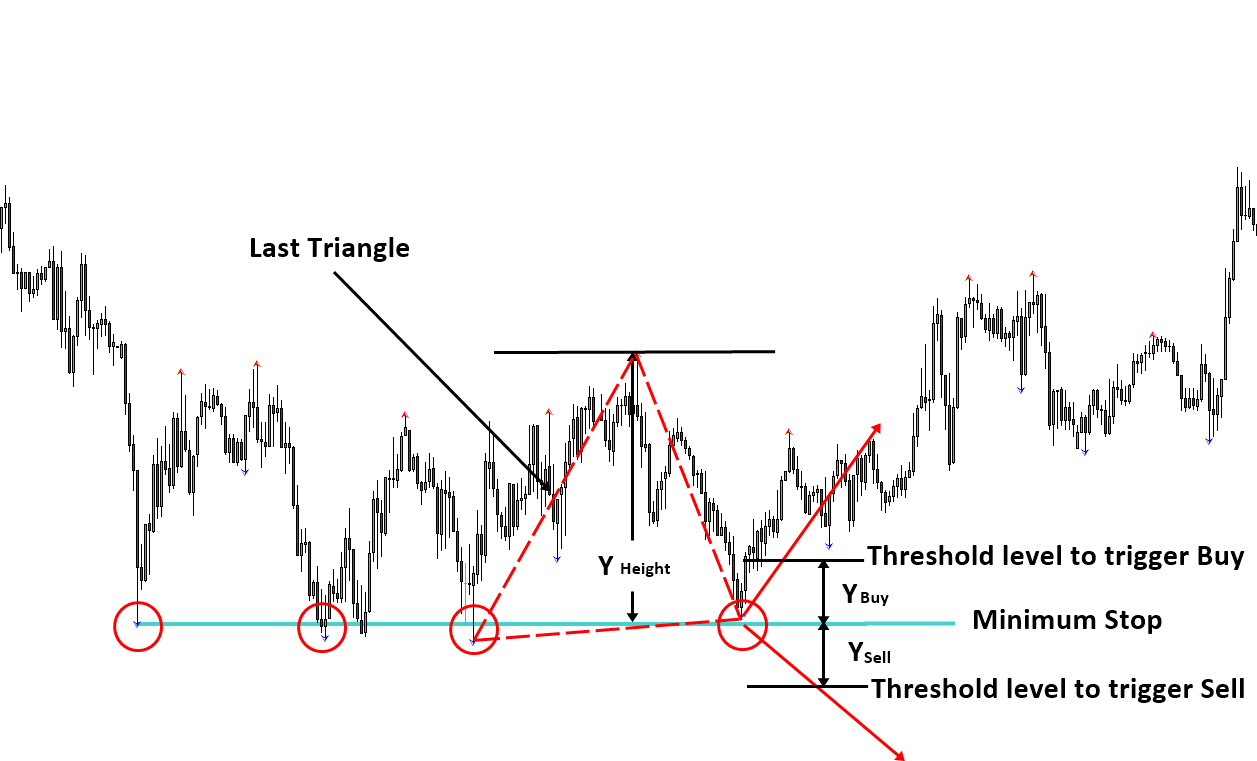# Horizontal Support and Resistance

Support and resistance techniques are one of the most popular trading strategy for many day traders. Support is the price level at which buying is stronger than selling. Hence, the declining of the price below the support levels is often refused. Typically, support level is located below the current market price. Likewise, resistance is the price level at which selling is stronger than buying. Hence, the rising of the price above the resistance level is often refused. Typically, the resistance level is located above the current market price of the instrument. In fact, several support and resistance techniques are the direct pattern recognition techniques to deal with the equilibrium fractal waves in the price series. However, trader should note that not all the support resistance techniques are the valid tool to deal with the equilibrium fractal waves. For example, the pivot levels derived from the pivot analysis can be used as support and resistance for your trading. In fact, many textbook will introduce the pivot analysis as the kind of the support and resistance tool. However, consider how to derive the pivot levels from the standard pivot calculation equations below:

• Pivot Point (P) = (High + Low + Close)/3
• Support 1 (S1) = (P x 2) – High
• Support 2 (S2) = P –  (High  –  Low)
• Resistance 1 (R1) = (P x 2) – Low
• Resistance 2 (R2) = P + (High –  Low)

, where High, Low and Close are the High, Low and Close of the previous candle bar.

As you can see, the median price, Pivot Point, of the previous candle bar is calculated first. Then the median price is used to derive the support and resistance 1 and 2. We can clearly see that this pivot analysis have nothing to do with any pattern recognition from your chart. It rather uses the concept of averaging and volatility from the centre of the pivot point. One can extend the standard pivot analysis using Fibonacci ratio. However, the extended pivot analysis is still not the direct pattern recognition either.

When we say the support and resistance as in the direct pattern recognition, we are talking about creating support and resistance levels by connecting peak to peak and by connecting trough to trough in your chart. For example, Figure 2-1 shows the support example on GBPUSD H4 timeframe. By connecting three troughs in the chart, we could identify the important support level and we can project the level for the next point for our trading. When the price was approaching to the support level fourth time, the price made a reversal (turning point) movement on the support level. This is what happened at least on the surface. However, what is exactly going on behind the scene? In fact, the support levels are the manifest of the propagation of the Equilibrium Fractal Wave through the price. To demonstrate the propagation of the Equilibrium Fractal Wave, we have added the Zig Zag line on each trough in Figure 2-1. When the price touches the support level each time, new triangle is added to the price wave. The price can continue like this making few more touches on the support level. Sometimes, it is common to see the support with five or more touches. In general, when the vertexes of several non-overlapping triangles are aligned through a straight line below the market price, the straight line becomes the support level.

In our first support example (Figure 2-1), we have visualized the reversal price action around the support.  However, it is possible that we can have the support predicting the breakout movement instead of the reversal. For example, the USDJPY in Figure 2-2 penetrated through the support making the breakout on its fourth touch. When the price breakout below the support level, we can see that the size of the last triangle was transformed to be bigger. This is the typical price action when the new equilibrium source is introduced to the market.Figure 2-1: Support example as in the direct pattern recognition on GBPUSD H4 timeframe.Figure 2-2: Support Example as in the direct pattern recognition on USDJPY H4 timeframe.

Now let us have a look at the resistance example. As before, we can identify the important resistance levels by connecting peak to peak. In Figure 2-3, we have connected three peaks to identify the resistance level. The price made a reversal (turning point) in its fourth touch on the resistance level. As in the support level, the resistance levels are formed when the vertexes of the several non-overlapping triangles are aligned near the same horizontal level. In this time, the horizontal levels will be located above the market price. As in the support example, it is possible to have a breakout through the resistance too. For example, the EURUSD in Figure 2-4 made a clean breakout on its seventh touch on the resistance level. In this example, the last triangle was transformed in its size as in the support example of Figure 2-2. By summarizing both support and resistances examples, we can identify four different horizontal support and resistance patterns for your trading as shown in Table 2-1.Figure 2-3: Resistance example as in the direct pattern recognition on GBPUSD H4 timeframe.Figure 2-4: Resistance example as in the direct pattern recognition on EURUSD H4 timeframe.

 Support and resistance Price Action Direction Example Horizontal Support Reversal Buy Figure 2-1 Horizontal Support Breakout Sell Figure 2-2 Horizontal Resistance Reversal Sell Figure 2-3 Horizontal Resistance Breakout Buy Figure 2-4

Table 2-1: List of horizontal support and resistance patterns for your trading.

Y Buy = Proportion (%) x Y Height     and

Y Sell = Proportion (%) x Y Height, where Y Height = the height of the last triangle and Proportion is fraction of the height of the last triangle expressed in percentage.

The typical proportion to calculate Y Buy and Y Sell can range from 5% to 20%. When you choose the proportion, you might have to consider few points. If you choose to use small proportion, then you might experience the false signal frequently. In addition, you might be suffering from the price pull back due to the tight stop loss. If you choose to use large proportion, then you might be reducing your reward/risk ratio. Therefore, you have to win more frequently to maintain your profits.

Another method to set the buy and sell threshold level is using the Fibonacci ratio. Again, the Fibonacci threshold level will be calculated in terms of the height of the last triangle. For example, Y Buy and Y Sell can be expressed using the following formula for Figure 2-5:

Y Buy = Fibonacci Ratio  x   Y Height     and

Y Sell = Fibonacci Ratio   x   Y Height, where Y Height = the height of the last triangle and Fibonacci ratio include the typical Fibonacci ratios like 0.236, 0.382, 0.500, and 0.618, etc.

Both the proportional threshold method and the Fibonacci threshold method are valid for your entry. You can pick up one between them according to your own preferences. Fibonacci threshold method tends to provide the entry with greater distance from the stop. Hence, it should be noted that the Fibonacci threshold method could reduce your rewards/risk ratio. However, if the size of the last triangle is too small, then you might prefer to use the Fibonacci threshold method because too tight stop loss can be hunted too easily. You can set your stop loss at the support or resistance level for your trading. However, it is possible that the price can come back to the support or resistance level for retesting. It is sensible to place your stop slightly greater than the support and resistance level. Hence, the support or resistance level can be only minimum stop for your trading. You should always set your stop loss slightly greater than the minimum stop level.Figure 2-5: Support Trading example as in the direct pattern recognition on GBPUSD H4 timeframe.

This article is the part taken from the draft version of the Book: Scientific Guide to Price Action and Pattern Trading (Wisdom of Trend, Cycle, and Fractal Wave). Full version of the book can be found from the link below:

You can also use Ace Supply Demand Zone Indicator in MetaTrader to accomplish your support resistance analysis. Ace Supply Demand Zone indicator is non repainting and non lagging indicator with a lot of powerful features built on. Especially, you can use it like the horizontal support and resistance for your trading as well as the direction prediction trading strategy.

https://www.mql5.com/en/market/product/40076

https://www.mql5.com/en/market/product/40075